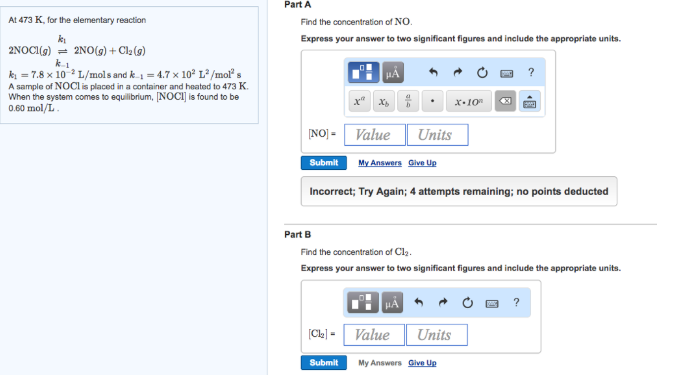# Problem: At 473 K, for the elementary reaction 2NOCl (g) k1k-1⇌ 2NO (g) + Cl2 (g)k1 = -7.8 x 10-2 L/mols and k-1 = 4.7 × 102 L2/mol2sA sample of NOCl is placed in a container and heated to 473 K. When the system comes to equilibrium, [NOCI] is found to be 0.60 mol/L.Part AFind the concentration of NO.Express your answer to two significant figures and include the appropriate units. Part B Find the concentration of Cl2. Express your answer to two significant figures and include the appropriate units.

###### FREE Expert Solution
90% (335 ratings)###### Problem Details

At 473 K, for the elementary reaction

2NOCl (g) k1k-1⇌ 2NO (g) + Cl2 (g)

k1 = -7.8 x 10-2 L/mols and k-1 = 4.7 × 102 L2/mol2s

A sample of NOCl is placed in a container and heated to 473 K. When the system comes to equilibrium, [NOCI] is found to be 0.60 mol/L.

Part A

Find the concentration of NO.

Express your answer to two significant figures and include the appropriate units.

Part B

Find the concentration of Cl2

Express your answer to two significant figures and include the appropriate units.